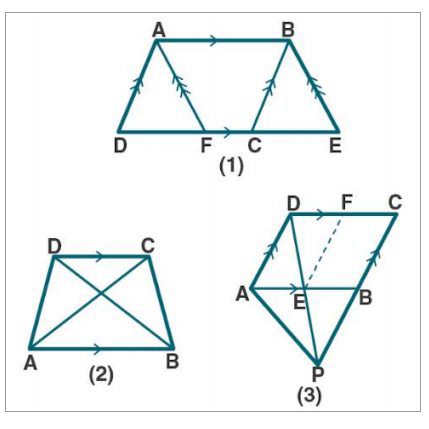# ML Aggarwal Solutions Class 9 Mathematics Solutions for Theorems on Area Exercise 14 in Chapter 14 - Theorems on Area

(a) In the figure (1) given below, the area of parallelogram ABCD is 29 \mathrm{cm}^{2}

Calculate the height of parallelogram ABEF if AB = 5.8 cm

(b) In figure (2) given below, the area of ∆ABD is 24 sq. units. If AB = 8 units, find

the height of ABC.

(c) In figure (3) given below, E and F are midpoints of sides AB and CD

respectively of parallelogram ABCD. If the area of parallelogram ABC is 36 \mathrm{cm}^{2}

(i) State the area of ∆ APD.

(ii) Name the parallelogram whose area is equal to the area of ∆ APD.(a) Given:

From fig (1)

ar ||gm ABCD = 29 \mathrm{cm}^{2}

To find:

Height of parallelogram ABEF if AB = 5.8 cm

Now, let us find

We know that ||gm ABCD and ||gm ABEF with equal bases and between the same

parallels so that their area is the same.

ar (||gm ABEF) = ar (||gm ABCD)

ar (||gm ABEF) = 29 \mathrm{cm}^{2} 2 ….. (1) [Since, ar ||gm ABCD = 29\mathrm{cm}^{2}

also, ar (||gm ABEF = base × height)

29 = AB × height [From (1)]

29 = 5.8 × height

Height = 29/5.8

= 5

∴ The height of parallelogram ABEF is 5cm

(b) Given:

From fig (2)

area of ∆ABD is 24 sq. units. AB = 8 units

To find:

Height of ABC

Now, let us find

We know that ar ∆ABD = 24 sq. units …… (1)

So, ar ∆ABD = ∆ABC ….. (2)

From (1) and (2)

ar ∆ABC = 24 sq. units

½ × AB × height = 24

½ × 8 × height = 24

4 × height = 24

Height = 24/4

= 6

∴ Height of ∆ABC = 6 sq. units

(c) Given:

From fig (3)

In ||gm ABCD, E, and F are midpoints of sides AB and CD respectively.

ar (||gm ABCD) = 36 \mathrm{cm}^{2}

From (1) and (2)

ar (∆ APD) = ½ × 36

= 18 \mathrm{cm}^{2}

(ii) we know that E and F are mid-points of AB and CD

In ∆CPD, EF || PC

Also, EF bisects the ||gm ABCD in two equal parts.

So, EF || AD and AE || DF

AEFD is a parallelogram.

ar (||gm AEFD) = ½ ar (||gm ABCD) ……. (3)

From (1) and (3)

ar (∆APD) = ar (||gm AEFD)

∴ AEFD is the required parallelogram which is equal to the area of ∆APD.

Related Questions
Exercises

Lido

Courses

Teachers

Book a Demo with us

Syllabus

Maths
CBSE
Maths
ICSE
Science
CBSE

Science
ICSE
English
CBSE
English
ICSE
Coding

Terms & Policies

Selina Question Bank

Maths
Physics
Biology

Allied Question Bank

Chemistry
Connect with us on social media!